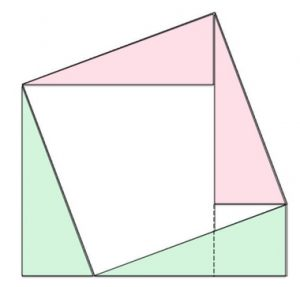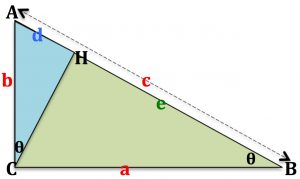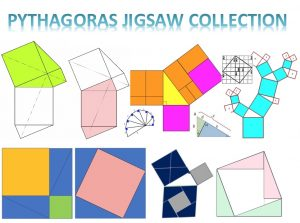There are hundreds of proofs of Pythagoras Theorem.

This diagram shows a proof.

Look carefully. Can you see three squares?

Assuming that they are squares, can you prove that four of the triangles are congruent?

Can you now write down a proof of Pythagoras’ Theorem and explain the proof?

The second diagram shows a well known proof using similar triangles.Angle ACB is a right angle and CH is perpendicular to AB.

Explain why the two angles labelled $\theta$ must be equal.

Explain why $b^2 = dc \quad (1)$.

Explain why $a^2 = ec \quad (2)$.

Combine equations (1) and (2) to give a proof of Pythagoras Theorem.The AIMSSEC Inclusion and Home Learning Guide has the theme JIGSAW Proofs. It provides jigsaw tasks suitable for all ages from 5 to 18+. The youngest learners can enjoy doing the jigsaws and learn from talking about the shapes and their properties. Older learners be asked to give explanations and proofs.